尊敬的用户：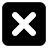三年级数学上册第四章《乘与除》整十整百整千数除以一位数的口算

• 同步课程
• 课后作业
• 同步试卷
• 本节重点
• 主讲老师

3×10=  6×50=  20×5=
12÷2=  14÷7=  36÷4=
6×40=  13×3=  23×4=

60÷2=30（根）
30×2=60  6÷2=3
6个十÷2=3个十
60÷2=30  60÷2=30

160根

160÷8=20（次）
80×2=160   16÷8=2
16个十÷8=2个十
160÷2=80  160÷8=20

2.算一算，说一说你是怎样想的。
60÷3=20  200÷5=40  240÷8=30  120÷4=30
560÷7=80 900÷3=300 210÷3=70  270÷9=30

42÷7=6
420÷7=60
4200÷7=600
35÷5=7
350÷5=70
3500÷5=700

25÷5=5
250÷5=50
2500÷5=500
25000÷5=5000

1.分给2人，平均每人....

2    80÷2=40
4    80÷4=20
8    80÷8=10

3.夺红旗。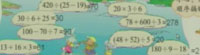4.这本书共180页。
（1）奇思用6天看完这本书，他平均每天看多少页？
（2）笑笑已经看了80页，剩下的要在5天内看完，平均每天要看多少页？
（3）再想一个生活中用（180-80）÷5解决的数学问题，与同伴说一说。
（1）180÷6=30（页）
（2）（180-80）÷5=100÷5=20（页）

一、填空题

1．直接写得数。
60÷3=     540÷9=    400÷5=    210÷7=
300÷6=    720÷8=    400÷2=    270÷3=

【参考答案】：20、60、80、30、50、90、200、90

二、主观题

1．想一想，填一填。

 × 20 50 70 × 10 2 3 30 4 5 300 6 9 7200

【参考答案】：
 × 20 50 70 × 10 60 800 2 40 100 140 3 30 180 2400 4 80 200 280 5 50 300 4000 6 120 300 420 9 90 540 7200

2．利民超市想采购80瓶饮料，需要买这样的多少捆？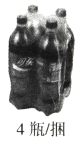【参考答案】：80÷4=20（捆）

3.1套儿童拼插地垫原价110元，优惠后每套比原来便宜多少元？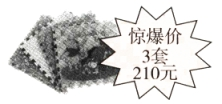【参考答案】：110-210÷3=40（元）

4．琪琪喜欢书法，她从星期一到星期三，一共写了120个汉字。照这样的速度，琪琪一个星期可以写多少个汉字？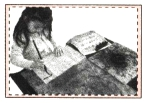【参考答案】：120÷3×7=280（个）

5．算一算，填一填，根据你观察到的规律比较出下面每组算式的大小。（☆和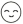都不为O）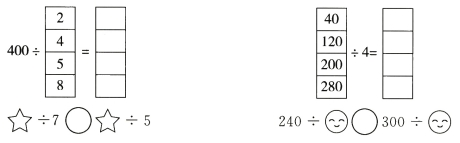【试题解析】：计算后从第一组题中可以发现，当被除数不变时，除数变大，商反而变小；从第二组题中可以发现，当除数不变时，被除数变大，商也变大。根据这些规律可以比较出☆÷7＜☆÷5，240÷＜300÷【参考答案】：200  100  80  50
10    30  50  70
＜  ＜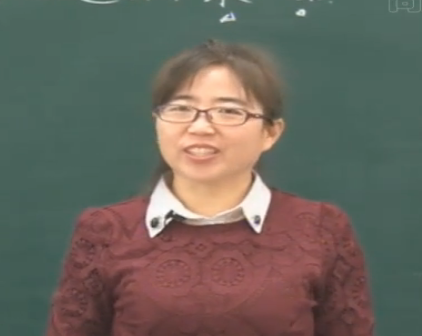[四川省成都市] 学着很轻松yehongli

2016-08-14 20:02:21